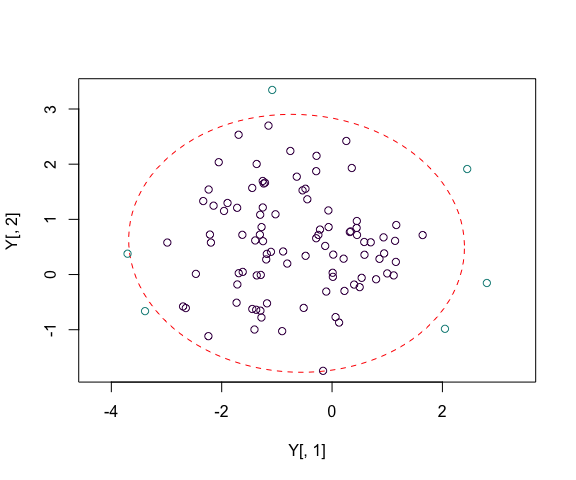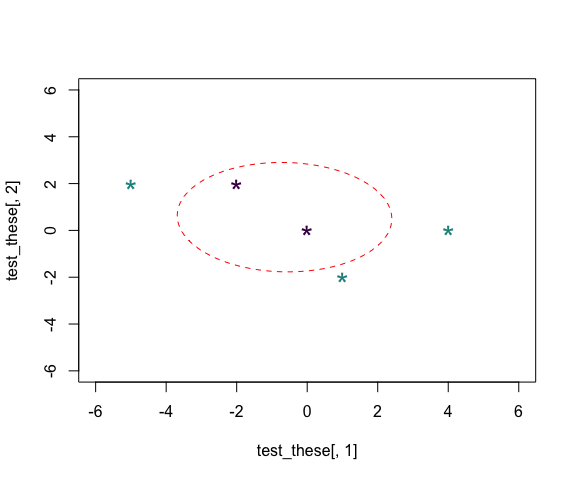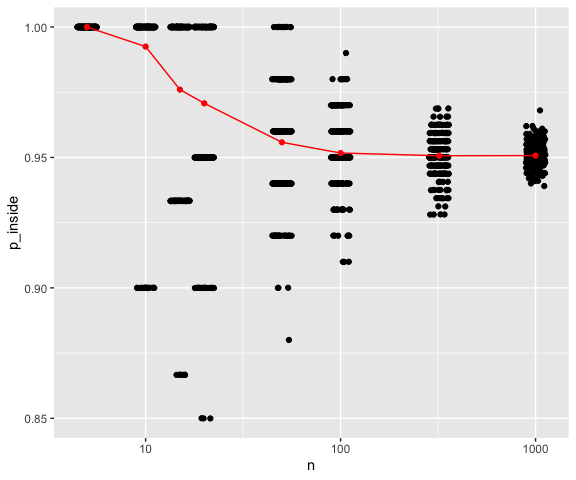# Points Inside or Outside Ellipse

## Creating some test data

First we will create some random data and draw an ellipse around it, and then we can test whether a defined set of other points are inside or outside the ellipse. This is done here by defining the seed for the random number generator to ensure we get the same data each time, but you may want to change this yourself to convince yourself that the method holds - with some uncertainty - around the expect proportion of points inside the ellipse. The function to set the random number generator is set.seed(3) and simply commenting this out will ensure new random numbers each time.


library(dplyr)
library(magrittr)
library(purrr)
#>
#> Attaching package: 'purrr'
#> The following object is masked from 'package:magrittr':
#>
#>     set_names
library(SIBER)
library(ggplot2)

# set the random seed generator so we get consistent results each time
# we run this code.
set.seed(3)

# n random numbers
n <- 100

# some random multivariate data
Y <- generateSiberGroup(n.obs = n)

## Determine whether points are inside or outside ellipse

Now we want to determined whether a set of data points are inside or outside our ellipse. This could be the same data as used to generate the ellipse, and given that its a 95% prediction ellipse, we would expect there to be 95% of the data inside the ellipse on average. As it happens, with this random seed, all our data points are inside the 95% ellipse: stochasticity is so random. It could though be a set of other independent data points. The way the code works below is a bit of space-warping trickery. Essentially, there is a transformation that can be applied to our ellipse that makes it a perfect circle, centred around the origin (point [0,0]). We apply this transformation to both the ellipse boundary and also our data points that we want to test. It is then very easy to determine whether or not our points are within the radius of our circle or whether they are outside! Moving the ellipse to the origin is easy, as it just means subtracting the mean of the data. Warping the ellipse so it maps onto a circle is done by a bit of linear matrix algebra using the covariance matrix of the data (the same that defines the ellipse). SIBER has functions to help do this.


# plot this example data with column 2 by column 1
# plot(Y[,2] ~ Y[,1], type = "n", asp = 1,
#      xlim = c(-10, 10),
#      ylim = c(-10, 10))

plot(Y[,2] ~ Y[,1], type = "n", asp = 1)

# add an ellipse, in this case a 95% ellipse
mu <- colMeans(Y) # centre of the ellipse
Sigma <- cov(Y) # covariance matrix of the ellipse

# percentile of the ellipse
p <- 0.95

# draw the ellipse
tmp <- addEllipse(mu, Sigma, p.interval = p, col = "red", lty = 2)

# Determine which of the samples are inside the ellipse
Z_samp <- pointsToEllipsoid(Y, Sigma, mu) # convert to circle space
inside_samp <- ellipseInOut(Z_samp, p = p) # test if inside

# inside points are marked TRUE which corresponds to 1 in numeric terms, and
# outside marked FALSE which corresponds to 0.
# So below I calculate (1 + !inside_test) which makes 1 correspond to inside
# and coloured black, and 2 correspond to outside and coloured red.
# and plot them with colour coding for whether they are inside or outside
points(Y[,2] ~ Y[,1], col = 1 + !inside_samp)Figure 1. The fitted 95% prediction ellipse with the samples coloured black if they are inside the ellipse and red otherwise. The expected proportion of points inside the ellipse is 0.95 and the observed proportion is 0.94.

# Define a matrix of 5 data points to test against our ellipse.
# For ease of interpretation of this code, I have built this matrix by
# specifying each row on a separate line and do this by adding the option
# byrow = FALSE (by default R fills down the rows first of a matrix).
test_these <- matrix(c(-2,  2,
0,  0,
-5,  2,
1, -2,
4,  0),
byrow = TRUE,
ncol = 2, nrow = 5)

# transform these points onto ellipsoid coordinates
Z_test <- pointsToEllipsoid(test_these, Sigma, mu)

# determine whther or not these points are inside or outside the ellipse drawn
# with same p as above (percentile).
inside_test <- ellipseInOut(Z_test, p = p)

# inside points are marked TRUE which corresponds to 1 in numeric terms, and
# outside marked FALSE which corresponds to 0.
# So below I calculate (1 + !inside_test) which makes 1 correspond to inside
# and coloured black, and 2 correspond to outside and coloured red.
# and plot them with colour coding for whether they are inside or outside
plot(test_these[,2] ~ test_these[,1],
col = 1 + !inside_test,
pch = "*",
cex = 2,
xlim = c(-6, 6),
ylim = c(-6, 6))

# and add the ellipse same as the one above
tmp <- addEllipse(mu, Sigma, p.interval = p, col = "red", lty = 2)Figure 2. A manual test of a few arbitrary points in space against the ellipse shown in Figure 1 just to satisfy ourselves that the process is working correctly.

## Large simulation across sample size

We can repeat the procedure above and assure ourselves that the 95% ellipses are behaving appropriately across a range of sample sizes. We first define a wrapper function that runs the above process with the number of samples $$n$$ as an input.


# a wrapper function to simulate a population, fit the ellipses and
# determine the proportion of samples inside.

testEllipse <- function(n, p) {

# generate the samples
Y <- generateSiberGroup(n.obs = n)

# sample moments
mu <- colMeans(Y) # centre of the ellipse
Sigma <- cov(Y) # covariance matrix of the ellipse

# Determine which of the samples are inside the ellipse
Z_samp <- pointsToEllipsoid(Y, Sigma, mu) # convert to circle space

# test if inside
inside_samp <- ellipseInOut(Z_samp, p = p)

# return propotion inside
return(sum(inside_samp) / length(inside_samp))

}

Set up the simulation over a range of sample sizes ($$n$$). The absolute minimum sample size is $$n = 3$$ in order to calculate a covariance matrix, but realistically since we are estimating two means and a covariance matrix then as we start to enter sample sizes $$n \lt 10$$ we start to run out of degrees of freedom pretty quickly.


# define the prediciton interval to use
prediction_interval <- 0.95

# specify a range of n
do_these_n <- c(5, 10, 15, 20, 50, 100, 320, 1000)

# how many replicates per n to simulate
reps_per_n <- 200

# replicate a range of n and sort on n
simExperiment <- tibble(n = rep(do_these_n, reps_per_n ),
p = prediction_interval) %>% arrange(n)

Loop over our experimental setup and add a column indicating the proportion of samples inside the fitted prediction ellipse.


simExperiment %<>% mutate(p_inside = pmap(list(n = n, p = p), testEllipse) %>% unlist)

# and summarise the results
summaries_simExperiment <- simExperiment %>% group_by(n) %>%
summarise(mu_p_inside = mean(p_inside),
min_p_inside = min(p_inside),
max_p_inside = max(p_inside))

And plot

# and plot p_inside against n
g3 <- ggplot(data = simExperiment, mapping = aes(x = n, y = p_inside)) +
geom_jitter() +
scale_x_log10() +
geom_point(data = summaries_simExperiment, mapping = aes(y = mu_p_inside),
color = "red") +
geom_path(data = summaries_simExperiment, mapping = aes(y = mu_p_inside),
color = "red")

print(g3)Figure 3. The effect of sample size on the proportion of samples falling inside the 95% prediction ellipse (black points - jittered) with the mean shown in red.

Clearly at large sample sizes we are getting very accurate and precise concordance between the simulated proportions of samples inside the 95% prediction ellipse with the predicted value at 0.95. The deviations from this become more pronounced and unstable $$\less approx 10$$. One of the main causes of this divergence is the integer nature of the samples. At $$n = 10$$ it is not possible to have exactly a proportion of 0.95 points inside the ellipse, and so the values tend towards either all 10 of them or 9 of them. There is increasing bias towards the ellipse containing more datapoints than expected as $$n \rightarrow 0$$.

## 3 and more dimensions

These functions will work just the same with more than 2 dimensions of data. In three dimensions you are testing whether your data are inside or outside a ball that has a transformation to and from an ellipsoid (spherical, cigar or frisbee shaped). The concept is exactly the same in 4 and more dimensions. The problem is illustrating the ellipsoid is not possible in >3 dimensions, and I have not yet implemented 3d versions of the ellipsoids, but I am sure many tutorials exist on how to plot these. Alternatively, and for illustrative purposes only you could plot each pair of your dimensions in turn, and add ellipses manually. The problem is that some points might appear to be in or out of the ellipse in these marginal plots, but might conflict with the true situation when all dimensions are considered simultaneously.

# set the random seed generator so we get consistent results each time
# we run this code.
set.seed(2)

# n random numbers
n_d <- 10^3

# number of dimensions
d <- 3

# vector of d means between -1 and +1
mu_d <- stats::runif(d, -1, +1)

# a (d x d) covariance matrix
# pull a precision matrix from the wishart distribution and invert it to
# get the corresponding covariance matrix.
sigma_d <- solve(matrix(stats::rWishart(1, d, diag(d)), d, d))

# n-dimensional multivariate random numbers for this test
Y_d <- mnormt::rmnorm(n_d, mu_d, sigma_d)

# sample mean and covariance matrix
mu_samp_d <- colMeans(Y_d) # centre of the ellipse
Sigma_samp_d <- cov(Y_d) # covariance matrix of the ellipse

# percentile of the ellipsoid to test
p <- 0.95

# here i am just going to test whether my actual data points are inside
# or outside the 95% ellipsoid but you could replace with your own
# data points as above

# transform these points onto ellipsoid coordinates
Z_d <- pointsToEllipsoid(Y_d, Sigma_samp_d, mu_d)

# determine whther or not these points are inside or outside the ellipse drawn
# with same p as above (percentile).
inside_d <- ellipseInOut(Z_d, p = p)

# how many of our points are inside our ellipse?
p_d_inside <- sum(inside_d) / length(inside_d)

The proportion of points inside our 3 dimensional ellipsoid is 0.95.